# High Level New Pattern Quantitative Aptitude Questions For IBPS Clerk Mains (Day-01) With Video Solutions

Dear Aspirants, Quantitative Aptitude plays a crucial role in Banking and all other competitive exams. To enrich your preparation, here we have provided New Pattern Aptitude Questions for IBPS Clerk Mains. Candidates those who are going to appear in IBPS Clerk Mains can practice these questions daily and make your preparation effective.

Note: Dear IG ‘ians, Once Again IBPS Guide Team has brought you here with a New Innovative, to make you understand the questions more easier we have started providing Video Solutions for the questions. Practice here & click the link given below to check the video solutions of the same questions. Make use of it & thanks for your love.

[WpProQuiz 4567]

### Click Below for Video Solutions for these Questions

Directions (1 – 5): Study the following graph carefully and answer the given questions.

The table shows the number of days taken by Swathi to complete the given percentage of work and the time ratio of Swathi to Shivani to complete the whole work.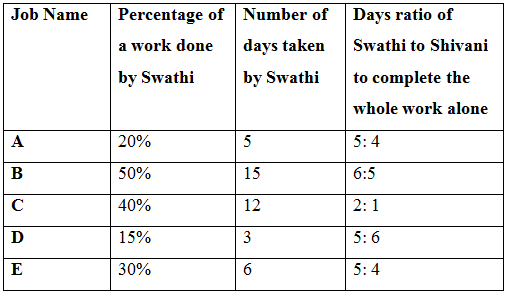1) Find the number of days taken by Sumaya to complete the Job-C alone?

Statement I: Swathi and Shivani started working to complete Job-C, Sumaya also joins with them to complete the work 4 days before the actual time taken by Swathi and Shivani working together.

Statement II: Swathi started working to complete Job-C with 75% of her original efficiency. After some days Sumaya joins with her and completed Job-C in 30 days. The ratio of the number of days taken by Swathi and Sumaya to complete Job-C is 2 : 1.

a) Only statement I alone is sufficient to answer

b) Only statement II alone is sufficient to answer

c) Either statement I or II alone is sufficient to answer the question

d) Both statements I and II alone are sufficient to answer the question

e) Both statements I and II alone are not sufficient to answer the question

2) Find the total wage to complete Job-B?

Statement I: Swathi and Shivani started working to complete Job-B with their 20% and 25% less than the original efficiency respectively. The ratio of the number of days taken by Swathi and Shivani to complete Job-B is 3 : 4. The difference between their wages is Rs. 300.

Statement II: Shivani started working to complete Job-B. After 6 days swathi also joins with her and increased her efficiency by 20%. Swathi left the work 5 days before the work was completed and get Rs. 8400 as wage.

a) Only statement I alone is sufficient to answer

b) Only statement II alone is sufficient to answer

c) Either statement I or II alone is sufficient to answer the question

d) Both statements I and II alone are sufficient to answer the question

e) Both statements I and II alone are not sufficient to answer the question

3) Find the total number of days taken to complete Job- A?

Statement I: Shivani started working to complete Job-A and after 5 days Janani joins with her. The ratio of the number of days they worked to complete Job-A is 35: 12.

Statement II: Sumi is 25% more efficient than Swathi to complete Job-A. They worked alternatively starting with Sumi to complete Job-A.

a) Only statement I alone is sufficient to answer

b) Only statement II alone is sufficient to answer

c) Either statement I or II alone is sufficient to answer the question

d) Both statements I and II alone are sufficient to answer the question

e) Both statements I and II alone are not sufficient to answer the question

4) Find the number of days taken by Krish alone to complete Job-D?

Statement I: Shivani and Krish started working to complete Job-D and the ratio of the number of days worked by Shivani to Krish is 5: 2.

Statement II: Shivani and Krish completes the Job- B in (375/88) days less than the total number of days taken by Shivani and Swathi working together till the work completed.

a) Only statement I alone is sufficient to answer

b) Only statement II alone is sufficient to answer

c) Either statement I or II alone is sufficient to answer the question

d) Both statements I and II alone are sufficient to answer the question

e) Both statements I and II alone are not sufficient to answer the question

5) What is the efficiency ratio of Janavi to Kamali?

Statement I: Shivani started working to complete Job- C and after 4 days, Janavi joins with him and after few days Janavi replaced by Kamali. The remaining work was completed in 5 days.

Statement II: Janavi and Kamali started working together to complete Job-B and completed the work same as the number of days taken by Shivani and Swathi working together.

a) Only statement I alone is sufficient to answer

b) Only statement II alone is sufficient to answer

c) Either statement I or II alone is sufficient to answer the question

d) Both statements I and II alone are sufficient to answer the question

e) Both statements I and II alone are not sufficient to answer the question

Directions (6 – 10): The questions below are based on the given Series-I. The series-I satisfy a certain pattern, follow the same pattern in Series-II and answer the questions given below.

6)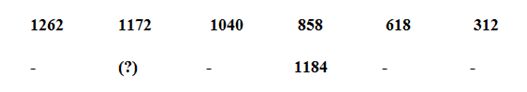a) 1288

b) 1326

c) 1498

d) 1452

e) 1374

7)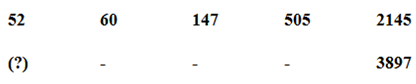a) 196

b) 125

c) 64

d) 133

e) 156

8)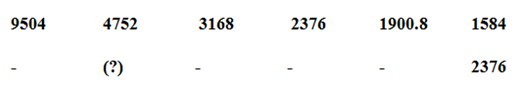a) 7128

b) 7456

c) 8421

d) 9632

e) 7568

9)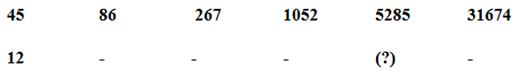a) 1254

b) 1321

c) 1692

d) 1471

e) 1325

10)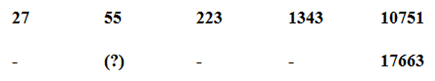a) 91

b) 84

c) 63

d) 109

e) 87

### Click Below for Video Solutions for these Questions

Directions (1-5):

Total number of days taken by Swathi to complete Job-A = 5/20*100 = 25 days

Total number of days taken by Shivani to complete Job-A = 25/5 *4 = 20 days

Total number of days taken by Swathi to complete Job-B = 15/50*100 = 30 days

Total number of days taken by Shivani to complete Job-B = 30/6 *5 = 25 days

Total number of days taken by Swathi to complete Job-C = 12/40*100 = 30 days

Total number of days taken by Shivani to complete Job-C = 30/2 *1 = 15 days

Total number of days taken by Swathi to complete Job-D =3/15*100 = 20 days

Total number of days taken by Shivani to complete Job-D = 20/5 *6 = 24 days

Total number of days taken by Swathi to complete Job-E = 6/30*100 = 20 days

Total number of days taken by Shivani to complete Job-E = 20/5 *4 = 16 days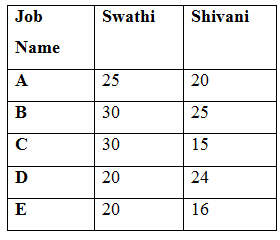Statement I: Swathi and Shivani started working to complete Job-C, Sumaya also joins with them to complete the work 4 days before the actual time taken by Swathi and Shivani working together.

LCM of 15 and 30 = 30

Total work = 30 units

Swathi = 1 unit

Shivani = 2 units

No. of days taken by Swathi and Shivani working together = 30/(1+2)

= 30/3 = 10 days

No. of days taken by Swathi, Shivani and Sumaya working together= 10 – 4

= 6 days

Swathi, Shivani and Sumaya work per day = 30/6 = 5 units

Sumaya work per day = 5 – 2 – 1 = 2 units

No. of days taken by Sumaya alone to complete the whole work = 30/2 = 15 days

Statement I alone is sufficient to answer the question.

Statement II: Swathi started working to complete Job-C with 75% of her original efficiency. After some days Sumaya joins with her and completed Job-C in 30 days. The ratio of the number of days taken by Swathi and Sumaya to complete Job-C is 2: 1.

Here there is no information about Sumaya’s one day work. From that we cannot answer the given question.

Statement II alone is not sufficient to answer the question.

Statement I: Swathi and Shivani started working to complete Job- B with their 20% and 25% less than the original efficiency respectively. The ratio of the number of days taken by Swathi and Shivani to complete Job-B is 3 : 4. The difference between their wages is Rs. 300

LCM of 25 and 30 = 150

Total work = 150 units

Swathi’s work per day = 5 units

Swathi’s 80% efficiency = 5 * 80/100 = 4 units

Shivani’s work per day = 6 units

Shivani’s 75% efficiency = 6 * 75/100 = 4.5 units

According to the question,

(3x * 4) + (4x*4.5) = 150

12x + 18x = 150

30x =150 => x =5

Number of days worked by Swathi and Shivani is 15 and 20 days respectively.

Swathi’s 15 days work = 4 * 15 = 60 units

Shivani’s 20 days work = 20 * 4.5 = 90 units

Difference between the total units done Shivani and Swathi = 90 – 60 = 30 units

Amount received to complete 30 units of total work = 300

Amount received to complete 150 units of total work = (300/30)*150 = Rs. 1500

Statement II: Shivani started working to complete Job-B. After 6 days swathi also joins with her and increased her efficiency by 20%. Swathi left the work 5 days before the work was completed and get Rs. 8400 as wage.

LCM of 25 and 30 = 150

Total work = 150 units

Shivani’s work per day = 6 units

Swathi’s work per day = 5 units

Swathi’s 120% efficiency = 5 * 120/100 = 6 units

Total number of days worked by Shivani be x and Swathi be (x – 11)

According to the question,

(x * 6) + ((x-11)*6) = 150

6x + 6x – 66 =150

12x = 216

X = 18 days

Total work done by Shivani = 6 * 18 = 108 units

Total work done by Swathi = (18 -11)*6 = 42 units

Amount received by Swathi to complete 42 units is 8400

Total amount to complete 150 units = 8400/42 * 150 = Rs. 30000

Statement I: Shivani started working to complete Job-A and after 5 days Janani joins with her. The ratio of the number of days they worked to complete Job-A is 35: 12.

Here, there is no information about Janani’s individual work. From that we cannot answer the given question.

Statement II: Sumi is 25% more efficient than Swathi to complete Job-A. They worked alternatively starting with Sumi to complete Job-A.

Efficiency ratio of Sumi to Swathi = 125: 100 = 5: 4

Days ratio of Sumi to Swathi = 4: 5

Number of days taken by Sumi alone to complete the whole work = (25/5) * 4

= 20 days

LCM of 25 and 20 = 100

Total work = 100 units

Swathi’s work per day = 100/25 = 4 units

Sumi’s work per day = 100/20 = 5 units

Work done by Swathi and Sumi 2 days = 9 units

Work done by Swathi and Sumi 22 days = 9*11= 99 units

Remaining = 100 – 99 = 1 unit

Required number of days = 22 + 1/5 = 22 (1/5) days

Statement I: Shivani and Krish started working to complete Job-D and the ratio of the number of days worked by Shivani to Krish is 5: 2.

Number of days worked by Shivani and Krish is 5x and 2x respectively.

Here, there is no information about Krish’s individual work. From that we cannot answer the given question.

Statement II: Shivani and Krish completes the Job- B in (375/88) days less than the total number of days taken by Shivani and Swathi working together till the work completed.

LCM of 30 and 25 = 150

Total work = 150 units

Swathi’s per day work = 5 units

Shivani’s per day work = 6 units

Total number of days taken by Swathi and Shivani to complete the whole work

= 150/11 days

Total number of days taken by Shivani and Krish to complete the whole work

= 150/11 – 375/88

= 825/88

Krish one day work = 88/875 – 1/25 = 11/165

Statement II alone is sufficient to answer the given question.

Statement I: Shivani started working to complete Job-C and after 4 days, Janavi joins with him and after few days Janavi replaced by Kamali. The remaining work was completed in 5 days.

From question there is no information about Kamali and Janavi alone to complete the whole work.

Statement I alone is not sufficient to answer the given question.

Statement II: Janavi and Kamali started working together to complete Job-B and completed the work same as the number of days taken by Shivani and Swathi working together.

From question there is no information about Kamali and Janavi alone to complete the whole work.

Statement II alone is not sufficient to answer the given question.

Direction (6-10) :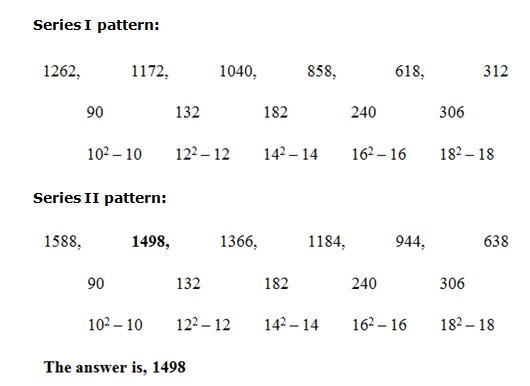Series I pattern:

52*1 + 23 = 60

60*2 + 33 = 147

147 *3 + 43 = 505

505*4 + 53 = 2145

Series II pattern:

125*1 + 23 = 133

133*2 + 33 = 293

293*3 + 43 = 943

943*4 + 53 = 3897

Series I pattern:

9504*(1/2) = 4752

4752*(2/3) = 3168

3168*(3/4) = 2376

2376*(4/5) = 1900.8

1900.8*(5/6) = 1584

Series II pattern:

14256*(1/2) = 7128

7128*(2/3) = 4752

4752*(3/4) = 3564

3564*(4/5) = 2851.2

2851.2*(5/6) = 2376

Series I pattern:

45*2 – 22 = 86

86*3 + 32 = 267

267*4 – 42 = 1052

1052*5 + 52 = 5285

5285*6 – 62 = 31674

Series II pattern:

12*2 – 22 = 20

20*3 + 32 = 69

69*4 – 42 = 260

260*5 + 52 = 1325

1325*6 – 62 = 7914

Series I pattern:

27*2 + 1 = 55

55*4 + 3 = 223

223*6 + 5 = 1343

1343*8 + 7 = 10751

Series II pattern:

45*2 + 1 = 91

91*4 + 3 = 367

367*6 + 5 = 2207

2207*8 + 7 = 17663